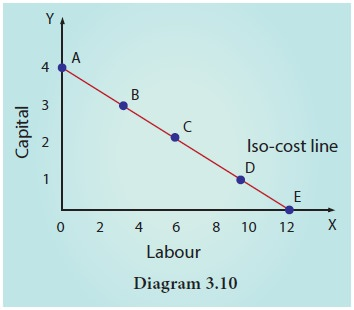Home | | Economics 11th std | The Iso-cost Line

# The Iso-cost Line

The iso-cost line is an important component in analysing producer’s behaviour.

The Iso-cost Line

The iso-cost line is an important component in analysing producer’s behaviour. The iso-cost line illustrates all the possible combinations of two factors that can be used at given costs and for a given producer’s budget. Simply stated, an iso-cost line represents different combinations of inputs which shows the same amount of cost. The iso- cost line gives information on factor prices and financial resources of the firm. It is otherwise called as “iso-price line” or “iso- income line” or “iso-expenditure line” or “total outlay curve”.Suppose that a producer has a total budget of  Rs.120 and for producing a certain level of output, he has to spend this amount on two factors Labour (L) and Capital (K). Prices of factors K is  Rs. 30 and L is  Rs.10. Iso Cost Curve can be drawn by using the following hypothetical table.

As shown in Table, there are five combinations of capital and labour such as combination A represents 4 units of capital and zero units of labour and this combination costs  Rs.120. Similarly other combinations (B,C,D and E) cost same amount of rupees ( Rs.120).

Symbolically,

4K + 0L=  Rs..120

3K + 3L=  Rs..120

2K + 6L=  Rs..120

1K + 9L=  Rs..120, and

0K + 12L=  Rs..120.

Thus, all the combinations A, B, C, D and E cost the same total expenditure.

From the figure 3.10, it is shown that the costs to be incurred on capital and labour are represented by the triangle OAE. The line AE is called as Iso-cost line.

Tags : Production Analysis | Economics , 11th Economics : Chapter 3 : Production Analysis
Study Material, Lecturing Notes, Assignment, Reference, Wiki description explanation, brief detail
11th Economics : Chapter 3 : Production Analysis : The Iso-cost Line | Production Analysis | Economics